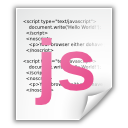# How To Convert Javascript Array To String

Hi Friends, In last article I have explained that how can we get checked check box values by jquery in array. Now if we want to convert that javascript array to string to use it in php So there is very simple way to do this. We can’t use js array in PHP as PHP array so we can convert it to string and then use that string in php.

Suppose for example exclude_category is javascript array which we want to convert in string to use it in PHP, So below is the way by which we can do it

`var arv = exclude_category.toString();`

toString is js function by which we can convert any js array to string. So now in arv we will get converted string from js array. let me know if you need any help in this.